# Brick weight

The brick weighs 2 kg and a half bricks.

How much does one brick weigh?

Correct result:

x =  4 kg

#### Solution: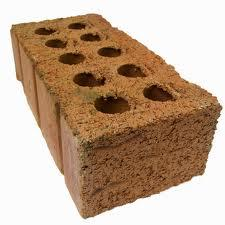We would be pleased if you find an error in the word problem, spelling mistakes, or inaccuracies and send it to us. Thank you!Math student
half bricks or half brick?
Or may be better, the weight of a half brick.Dr Math
we updated spelling of this math problem, thank you!Math student
needs rephrasingTips to related online calculators
Do you have a linear equation or system of equations and looking for its solution? Or do you have quadratic equation?

## Next similar math problems:

• GrandmaWe're going to Grandma. We still have 24 km left. How far does Grandma live if we have traveled from the total distance: half, a third, a fifth?
• Worker salaryThe worker had a salary of CZK 18,000. During the year, his salary was increased by a quarter. He earned a total of CZK 247,500 for the whole year. From which month was his salary increased?
• The sumThe sum of five consecutive odd numbers is 75. Find out the sum of the second and fourth of them.
• Second sideCalculate the length of the other side of the rectangle if its circumference is 60 cm and one side is 10 cm long.
• LibraryNew books were purchased for the library. Five-eighths were professional books, one-fifth were encyclopedias, and 231 books were dictionaries. How many professional books were there?
• Equation with one variableSolve the following equation with one unknown: 5(7s + 5) =130
• The temperature 4The temperature was very cold, then it doubled, then it dropped 10 degrees, and then it increased by 40 degrees. The temperature is now 16 degrees. What was the starting temperature?
• The orchard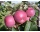4 temporary workers harvested the orchard in 9 days. How many temporary workers we need for six days?
• Equal temperature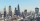The temperatures of the two cities were measured at the same time. The temperature in city A was 60 degrees And rose at a constant rate of 2 degrees per hour. The temperature in city B was 40° and rose at a constant rate of 10° per hour Enter the time in
• Pupil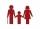I'm a primary school pupil. I attended the exercises of parents with children 1/4 of my age, 1/3 for drawing, and 1/6 for flute. For the first three years of my life, I had no ring, and I never went to two rings at the same time. How old am I?
• The tourist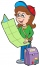The tourist has walked 3/4 of the route. The destination is 12.5 km away. How many kilometers did the route measure?
• Father and sons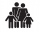Father is 27 and his sons 2 and one year. In how many years will his sons sum up to half his age?
• Simple equation 1035= 7*3*x what is x?
• 45th birthdayThis year Mrs. Clever celebrated her 45th birthday. Her three children are now 7, 11 and 15 years old. In how many years will Mrs. Clever's age be equal to the sum of her children's years?The fruit basket is five times heavier than the empty basket. Filled is 20kg heavier than empty. Determine the weight of the fruit in the basket.Calculate the radius of the circle whose length is 107 cm larger than its diameterVilem, Cenek, and Edita divided the money they earned by spreading the leaflet. Vilem got 240 CZK more than Cenek and twice more than Edita. Edita got 400kc less than Vilem.